• ms@ms.lt
• +370 607 27 665
• My work is in the Public Domain for all to share freely.

Introduction E9F5FC

Understandable FFFFFF

Questions FFFFC0

Notes EEEEEE

Software

I am studying the classical Lie groups and algebras.

In particular, I am investigating, Why, intuitively, are there four classical Lie groups/algebras?

Study the symmetry inherent in math

• Consider the ways of reflection across matrices: up or down, left or right, diagonal or anti-diagonal, and the combinations. For example: down/up then across-the-diagonal (=transpose) then down/up = across-the-anti-diagonal. Note how this breaks up the matrix into 2x2 compartments. And consider why we might assign minus signs. Note that an arbitrary matrix codes for an arbitrary quadratic form. And from this perspective interpret all of the definitions of specific Lie groups, Lie algebras and quadratic forms.
• For {$B_n$}, {$C_n$}, {$D_n$} since there are four elements on the diagonal, there are two kinds of pairs involved. Diagonal elements are paired by an eigenvector, but also the diagonal elements come in positive and negative pairs. This means that an eigenvector yields a pair of pairs. An eigenvector is a pair of bases which each accord with a pair of diagonal elements. {$B_n$}, {$C_n$}, {$D_n$} give the options for relating that pair of pairs. What are those options?
• relation between spin (half-integer and integer) and statistics (Fermion and Boson) and the metaphysics of the latter regarding distinguishability

Understand the underlying Lie theory

• Simplicity is, for my purposes, an artificial construct - it's not necessarily a problem that U(n+1) is not simple (see: intuitive explanation. Note also that one-dimensional abelian Lie algebras are not considered simple - is that perhaps a contrivance?

Make Lie algebras concrete

• Eigenvalues {$e_i$} are related to {$a_{ii}$} as {$a_{ij}=0$}. Study these types of issues.
• How do root systems relate two different inputs: the eigenvalue and the eigenvector?
• Write out Serre's relations for each of the classical Lie algebras.

Root as perspective

• A root relates two dimensions. A perspective relates two scopes.
• Imagine the ways of extending a perspective and not running into trouble, not collapsing a perspective. What is a perspective?

Identify key constraints on root systems and work backwards from them

• {$4 \textrm{cos}^2\theta$} is an integer
• the Cartan matrix
• How the constraints on the Cartan matrix make for three kinds of reversals.
• What it means for a propagating signal to branch and how this yields the other exceptional groups.
• Constraint on -A.
• Interpret G2 as a reflection of a signal. Why can't it come after propagation?
• Make sense of all of the exceptional groups including F4.
• Why is it important that the Cartan matrix be invertible? S positive definite?
• How is the Cartan matrix related to the matrix defined by the orthogonal basis underlying the fundamental roots? Or to the linear logical equations that result from the equation xT S T = 0?
• Intuitively, why is the Cartan matrix not invertible if the chain is a loop? or has B, C, D type endings at both ends? In what sense is a signal propagated across the entire chain? Is it the propagation of slack?
• Do the chains relate to chains of set inclusion and the problems of extending them? (no cycles, etc. all the ways things can go wrong?)
• Suppose the chain is a broken loop! (Or has its node at infinity?) Can it be recovered, extended, etc.?
• the Dynkin diagram
• Can consider how to summarize the constraints on the Dynkin diagrams. Ways to expand from one node. From two linked nodes. And so on.
• Can we have a Dynkin diagram (Cartan matrix) where two nodes are linked by arrows (steps) in both directions? But that is what we have.
• Imagine how the root systems for the A, B, C and D series complete the A chain.
• Imagine how 3 series, how 3 dimensions come together in one dimension.
• Why can't 4 or more dimensions come together in one dimension?
• Consider the algebraic meaning of the various connections between dimensions. How do they relate to the Lie bracket? And to transpositions? Defining the composition of group elements and also their inverses?
• Study the augmentation of Dynkin diagrams, how that relates to the negative of the maximal root, what it means to add that negative maximal root, what kind of degeneracy results, and how it relates to the constraints on the Lie algebra, for example, that the trace is zero.
• The constraints provided by an infinite number of conditions, such as an infinite number of orders of magnitude, seem to drive very strict forms of parity. What is going on?
• What constrains a root system? so that it is not the whole root lattice?
• The characteristic polynomial {$\det (\mathrm{ad}_L x - t)$} whose roots are the roots.

Explore basic concepts

• What are the bilinear forms and quadratic forms for Lie groups/algebras.
• complementary relationships between elements and bilinear forms as regards symmetric vs. skew-symmetric
• The pseudoscalar as the totality. How does it relate to Clifford algebras and to polytopes and the element of highest weight in root systems? And the duality between (implicit) center and (explicit) totality? And how is its fourfold pattern driving Bott periodicity?
• Think of an eigenvalue as an anti-sum of the rest and then how that anti-sum collapses further. How it breaks down into 0. And how the breakdown will assemble?
• Relate the simple roots and negative maximal root.
• Consider the distance from simple root to its reflection.

Relate root systems and polytopes

• Study their Weyl groups.
• Consider the Weyl group generated by reflections of the root space.
• What happens intuitively when we augment the simple roots by the negative maximal root? What kind of degeneracy does that yield?
• What is the natural way to relate Weyl groups to polytopes based on the root systems?
• What kind of polytopes do the Weyl chambers form out of a sphere?
• Relate polytopes to the simple roots and the negative maximal root.
• How does the unfolding of root systems (with their propagation and reflection of signals) relate to the unfolding of polytopes?
• How is the mirror central to the construction of cross-polytopes (pairs of vertices), hypercubes (mirrors) and demihypercubes (halves)?

Interpret Lie algebras

• in interpreting the root system of {$A_n$}, find an interpretation of the constraint on the eigenvalues of H for {$A_n$} that their sum have zero trace
• Note that for {$B_n$}, {$C_n$}, {$D_n$}, there are basically two kinds of roots ei-ej and ei+ej. Describe the ei+ej roots in a way that is universal for all three families. And these two sets of roots are linked by an additional simple root in three possible ways. Count these root systems. Do they add up to a square (two staircases? like a square matrix? thus a quadratic form?) perhaps minus one element?
• For Lie algebras, consider how to think of an arbitrary element as a combination of basis elements and thus as weights on a simplex (given by the basis elements). Then basically we are dealing with the possible relationships with pairs of basis elements but this can be expanded to consider larger sets. And we are dealing with "halves" of basis elements in that an eigenvector typically consists of a pair of basis elements.
• Consider also that {$B_n$}, {$C_n$}, {$D_n$} may be thought of as the ways of relating a pair of simplexes. And relate that to the polytope families, which should manifest those same relationships.
• Interpret the Lie product as vector subtraction. Subtraction is nonassociative and this introduces a duality between substraction of a sum and substraction of an alternating sum. Is this perhaps related to the Koblantz conjecture?
• Define the positive definite matrix S intuitively. Interpret its constraints in terms of systems of simple equations. Relate those equations to Dynkin diagrams.

Relate the different kinds of numbers

• The Fundamental Theorem of Algebra is related to the closeness of the complexes to the reals. So study the theorem's proofs accordingly. For example, the winding number proof seems to be about parity between inside and outside. Other proofs seem to be about the parity between odd and even powers. (Odd powers must clearly have a root.) So the crucial case is an even power that is above the x-axis. Can you construct a proof where you add a negative constant to lower it below the x-axis? In which case you get real roots? Also consider how the orders of magnitude ripple out, each becoming dominant at some point compared to the smaller ones.

Relate to symmetric functions of eigenvalues

• Consider the Lie groups and Lie algebras in terms of constraints on symmetric functions of the eigenvalues of the matrices. For example, the trace is 0 or the determinant is 1.
• Express each Lie group and Lie algebra in terms of constraints on symmetric functions of eigenvalues.
• If the determinant is 1, then what are the implications for Cramer's rule?

Study the geometry of the Lie groups.

• How does geometry come into play with the Lie groups? How do the different kinds of geometry arise and distinguish themselves?
• How is geometry the regularity of choice?
• How is geometry related to preserving the inner product?
• Understand how the inner product is preserved: complex, real, quaternionic.
• Investigate the quality preserved: the relation to the affine, projective, conformal and symplectic geometries.
• How are inner products and quadratic forms related?
• How do symplectic matrices preserve oriented areas?
• What do the constraints on reversal mean in terms of Lie groups and binormal forms? What dictates such constraints?

Understand Clifford theory

Understand Bott periodicity

• Understand the Artin-Wedderburn theorem, starting with Wedderburn's original result.
• Where have I encountered the fourfold periodicity for the shuffle permutation?

I am trying to understand Lie groups and algebras because they are central to all of mathematics. Also, it seems that the four classical Lie groups/algebras describe four basic geometries in my diagram of ways of figuring things out.

Duality of element and its inverse. Assuring nice inverses

Lie groups are continuous groups. A group involves an inherent duality between an element and its inverse by which every expression has a dual expression in terms of inverses. We can think of the element-inverse duality as an "internal" duality as if one was inverting a shirt from the outside to the inside. Related to this duality is an "external" duality where we can read every expression from left to right or from right to left. This latter duality is external in that it is a duality of form, whereas the internal duality is a duality of content.

A continuous group expresses a continuous duality which can be understood in terms of infinitesimals. This duality is therefore expressed in terms of continuous parameters, thus in terms of divisions rings such as the real numbers, complex numbers, or quaternions. If we restrict ourselves to a finite number of generators, then we can represent the group elements with matrices. Inverses of matrices are given by Cramer's rule. Now, Cramer's rule defines elements in terms of fractions. If we want to deal with integer matrices (why?) then each denominator needs to go away, which means that the matrix must be positive-definite (?) The end result is that the rule for inversion becomes very straightforward, such as transposition (prove). And the issue becomes how to define inversion, how to define that duality... For complex numbers, that duality is intrinsic.

Thinking in pairs of dimensions

It seems that it is all about how to think in pairs of dimensions.

• Dualities express the pairs in all manner of ways.
• The complex numbers are central.
• Rotations occur in two dimensions and relate them.
• Spinors relate two dimensions even more tightly than rotations.
• The matrix {$J$} important for symplectic matrices is just the determinant of the 2 dimensional matrix, the area of a parallelogram: {$a_{11}a_{22} - a_{12}a_{21}$}.
• A pair of real numbers may act like two independent spaces like the split endpoints of {$D_n$}.

Propagating and reflecting a signal for counting

• The simple roots are the ways of propagating a signal, which is to say, of sending it and receiving it. By default, the signal is propagated outward by ever linking to a new node. But the signal can invert itself in three ways: by linking to zero, a mirror which will link to its reflection; by linking directly to its own reflection; or by itself serving as a mirror and linking to the reflection of the previous node. In the latter case, the node is implicitly identified its own reflection but is not explicitly equal to it! (For explicit equality Xn - Xn yields 0.) Thus these cases consider the differences between implicit identification and explicit equality.
• Reflected sequence can't be infinite but must halt. It is counting top-down rather than bottom-up.

Concepts

Bilinear form

• A bilinear form B : V × V → K is called reflexive if B(v, w) = 0 implies B(w, v) = 0 for all v, w in V.
• A bilinear form B is reflexive if and only if it is either symmetric or alternating.

Root systems and polytopes

The hyperplanes of root systems create Weyl chambers and form their edges. Each Weyl chamber is a face of a polytope.

Root system as a pair of spheres

Each of the four classical root systems can be thought of as relating two or three spheres, which is to say, two or three sets of roots {$S_r$}, where the roots in {$S_r$} all have the same length. I distinguish between end roots such as {$\pm e_i$} and {$\pm 2e_i$} and main roots such as {$\pm (e_i \pm e_j)$}. The main roots consist of two spheres of the same radius, Plus and Minus, dictated by the inner sign, which are taken to be the same in the case of {$A_n$} and different otherwise.

• {$A_n$} Plus sphere and Minus sphere are the same (beyond system)
• {$B_n$} end root sphere inside the main root sphere (looking-stepping into system)
• {$C_n$} main root sphere inside the end root sphere (looking-stepping out of system)
• {$D_n$} Plus sphere and Minus sphere are different (within system)

Perhaps we may think of the Plus sphere and Minus sphere having positive and negative radius, accordingly. And perhaps the two spheres are the same in the case of {$A_n$} but distinct otherwise. We may think of {$B_n$} and {$C_n$} as intermediary between same and different. Note that they are looking at opposite directions and so their spheres are inverted. This means that the end root sphere is the system's sphere. Consequently, outide the end root sphere there is no distinction between Plus and Minus and they function completely in parallel. Whereas inside end root sphere they are expressed differently with regard to the end roots.

Note that there is, additionally, a zero sphere.

Similarly, the polytopes can be thought of as generated by trinomials. (I then need to interpret as trinomials the binomials for the simplexes and the coordinate systems.)

Differences between odd and even orthogonal matrices

Conformal orthogonal group Being isometries, real orthogonal transforms preserve angles, and are thus conformal maps, though not all conformal linear transforms are orthogonal. The group of conformal linear maps of Rn is denoted CO(n) for the conformal orthogonal group, and consists of the product of the orthogonal group with the group of dilations. If n is odd, these two subgroups do not intersect, and they are a direct product: CO(2k + 1) = O(2k + 1) × R∗, where R∗ = R∖{0} is the real multiplicative group, while if n is even, these subgroups intersect in ±1, so this is not a direct product, but it is a direct product with the subgroup of dilation by a positive scalar: CO(2k) = O(2k) × R+.

Cayley-Dickson construction for pairs of numbers

• Complex 2 dimensions (dvejybė) leads to quaternions 4 (likimas) and two copies of the reals 1+1 (laisva valia).
• quaternions split the commutativity of the complex numbers

Lie groups and Lie algebras

• Derivative of a curve through the point t=1 is the formulation that links Lie groups and Lie algebras.
• All symmetry groups are subgroups of the orthogonal group.

Symmetric functions of eigenvalues of a matrix

Supposing there is a diagonal matrix whose entries are all distinct, then the subspace of all diagonal matrices is a Cartan subalgebra.

The classical Lie groups and Lie algebras: Table of Properties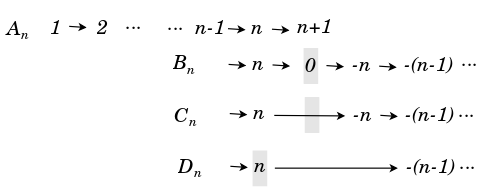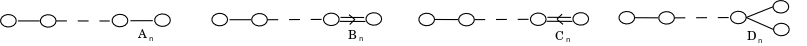family {$A_n$} {$B_n$} {$C_n$} {$D_n$} Lie algebra {$\mathfrak {sl}_{n+1}$} {$\mathfrak{so}_{2n+1}$} {$\mathfrak {sp}_{2n}$} {$\mathfrak{so}_{2n}$} dimension ... odd 2n+1 even 2n even 2n Lie algebra special linear special orthogonal symplectic special orthogonal nondegenerate bilinear form symmetric skew-symmetric symmetric constraints on element as matrix A vanishing trace skew-symmetric {$-A=A^{T}$} {$-A=\Omega^{-1}A^\mathrm{T} \Omega$} skew-symmetric {$-A=A^{T}$} intrinsic definition sums of simple bivectors (2-blades) v ∧ w sums of simple bivectors (2-blades) v ∧ w symmetric function of eigenvalues {$w_{1}+...+w_{n}=0$} Cartan subalgebra {$h_{1},h_{2}...h_{n-1},$} {$h_{1}...h_{n},0,$} {$h_{1}...h_{n},$} {$h_{1}...h_{n},$} diagonal entries {$-h_{1}-h_{2}-...-h_{n-1}$} {$-h_{1},...,-h_{n-1}$} {$-h_{1},...,-h_{n-1}$} {$-h_{1},...,-h_{n-1}$} constraint from matrix i≠j i≠j root length all equal one root shorter than the rest one root longer than the rest all equal nth simple root given simple roots {$x_{i}-x_{j}$} {$x_{n}-x_{n+1}$} {$x_{n}-0$} {$x_{n}--x_{n}$} {$x_{n}--x_{n-1}$} roots beyond {$\pm (x_i-x_j)$} {$\pm (x_i+x_j), \pm x_i$} {$\pm (x_i+x_j), \pm 2x_i$} {$\pm (x_i+x_j), i\neq j$} sequence of basis elements {$x_{1},\dots,x_{n},x_{n+1},\dots$} {$\dots,x_{n},0,-x_{n},\dots$} {$\dots,x_{n},-x_{n},\dots$} {$\dots,x_{n-1},x_{n},-x_{n-1}\dots$} duality - a total of 8 dimensions! two dimensions: forward outside and backward inside one dimension - outside - collapsed four dimensions - two sequences one dimension - inside - collapsed temporal interpretation future absolute present past ultimate node: the bridge to self-duality new node: no reflection zero reflection of same node reflection of previous node signal behavior propagates outward, away from its reflection links to zero, a mirror which will link to its reflection links directly to its own reflection identifies with its own reflection, thus serves as a mirror and links to the reflection of the previous node distance from its own reflection ≥2 2 1 0 nature of mirror no mirror explicit mirror implicit mirror self-mirror Lie group special unitary SU(n) special orthogonal SO(2n+1) symplectic Sp(2n, C) special orthogonal SO(2n) group elements as matrices unitary with determinant 1 orthogonal: columns and rows are orthonormal symplectic with entries in C orthogonal: columns and rows are orthonormal inverse matrix equals conjugate transpose transpose quaternionic transpose transpose group preserves volume and orientation in {$\mathbb{R}^{n}$} distance and a fixed point oriented area? distance and a fixed point related group preserves inner product U(n): complex O(n):real Sp(n):quaternionic O(n):real generalization of group preserves nondegenerate bilinear form or quadratic form - symmetric skew-symmetric symmetric Weyl group / Coxeter group symmetric group hyperoctahedral group hyperoctahedral group subgroup of index 2 of the hyperoctahedral group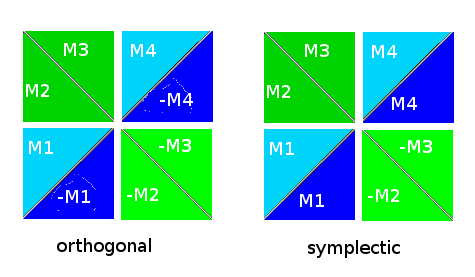Cartan matrix

The Cartan matrix is determined by the inner product as shown below. It gives the number of times one root my be added to another root and stay within the root system. Thus it is a measure of slack or freedom. This means that {$G_{2}$} measures a three fold slack of the kind needed for the operations +1, +2 and +3 on the eight-cycle of divisions of everything.

{$a_{ij}= \frac{2(r_{i},r_{j})}{(r_{i},r_{i})}$}

H, X and Y are determined by Serre's relations.

 Lie family Cartan matrix {$H_{i}$} {$X_{i}$} {$Y_{i}$}

{$A_{n} \begin{bmatrix} \ddots & & \\ & 2 & -1 \\ & -1 & 2 \end{bmatrix}$}

{$\begin{pmatrix} \ddots & & \\ & 1 & \\ & & -1 \end{pmatrix}$} {$\begin{pmatrix} \ddots & & \\ & 0 & 1\\ & & 0 \end{pmatrix}$} {$\begin{pmatrix} \ddots & & \\ & 0 & \\ & 1 & 0 \end{pmatrix}$}

{$B_{n} \begin{bmatrix} \ddots & & \\ & 2 & -2 \\ & -1 & 2 \end{bmatrix}$}

Almost all of the simple roots look like this... And they generate a first set of roots (as with the {$A_{n}$} family).

{$\begin{pmatrix} 1 & & & & \\ & -1 & & & \\ & & \ddots & & \\ & & & {\color{Red} 1} & \\ & & & & {\color{Red}{-1}} \end{pmatrix}$} {$\begin{pmatrix} 0 & 1 & & & \\ & 0 & & & \\ & & \ddots & & \\ & & & 0 & {\color{Red}{-1}}\\ & & & & 0 \end{pmatrix}$} {$\begin{pmatrix} 0 & & & & \\1 & 0 & & & \\ & & \ddots & & \\ & & & 0 & \\ & & & {\color{Red}{-1}} & 0 \end{pmatrix}$}

And there is one more below. (What type of interference will you have if you count forwards and backwards at the same time? Here they superimpose to give 0.)

{$\begin{pmatrix} 0 & & & & \\ & 1 & & & \\ & & -1 + {\color{Red}{1}} & & \\ & & & {\color{Red}{-1}} & \\ & & & & 0 \end{pmatrix}$} {$\begin{pmatrix} 0 & & & & \\ & 0 & 1 & & \\ & & 0 & {\color{Red}{-1}} & \\ & & & 0 & \\ & & & & 0 \end{pmatrix}$} {$\begin{pmatrix} 0 & & & & \\ & 0 & & & \\ & 1 & 0 & & \\ & & {\color{Red}{-1}} & 0 & \\ & & & & 0 \end{pmatrix}$}

And together this generates a second set of roots (in the other quadrants) which look like this...

{$\begin{pmatrix} 1 & & & & \\ & {\color{Red}{1}} & & & \\ & & \ddots & & \\ & & & -1 & \\ & & & & {\color{Red}{-1}} \end{pmatrix}$} {$\begin{pmatrix} & & & 1 & 0 \\ & & & 0 & {\color{Red}{-1}} \\ & & 0 & & \\ & 0 & & & \\ 0 & & & & \end{pmatrix}$} {$\begin{pmatrix} & & & & 0 \\ & & & 0 & \\ & & 0 & & \\ 1 & 0 & & & \\ 0 & {\color{Red}{-1}} & & & \end{pmatrix}$}

{$C_{n} \begin{bmatrix} \ddots & & \\ & 2 & -1 \\ & -2 & 2 \end{bmatrix}$}

{$\begin{pmatrix} 1 & & & & \\ & -1 & & & \\ & & \ddots & & \\ & & & {\color{Red} 1} & \\ & & & & {\color{Red}{-1}} \end{pmatrix}$} {$\begin{pmatrix} 0 & 1 & & & \\ & 0 & & & \\ & & \ddots & & \\ & & & 0 & {\color{Red}{-1}}\\ & & & & 0 \end{pmatrix}$} {$\begin{pmatrix} 0 & & & & \\1 & 0 & & & \\ & & \ddots & & \\ & & & 0 & \\ & & & {\color{Red}{-1}} & 0 \end{pmatrix}$}

Here they superimpose to give a mixed state {$\pm 1$}.

{$\begin{pmatrix} \ddots & & & \\ & 1 & & \\ & & -1 & \\ & & & \ddots \end{pmatrix}$} {$\begin{pmatrix} \ddots & & & \\ & 0 & \pm 1 & \\ & & 0 & \\ & & & \ddots \end{pmatrix}$} {$\begin{pmatrix} \ddots & & & \\ & 0 & & \\ & \pm 1 & 0 & \\ & & & \ddots \end{pmatrix}$}

The second set of roots look like this:

{$\begin{pmatrix} 1 & & & & \\ & {\color{Red}{1}} & & & \\ & & \ddots & & \\ & & & -1 & \\ & & & & {\color{Red}{-1}} \end{pmatrix}$} {$\begin{pmatrix} & & & \pm 1 & 0 \\ & & & 0 & {\color{Red}{\pm 1}} \\ & & 0 & & \\ & 0 & & & \\ 0 & & & & \end{pmatrix}$} {$\begin{pmatrix} & & & & 0 \\ & & & 0 & \\ & & 0 & & \\ \pm 1 & 0 & & & \\ 0 & {\color{Red}{\pm 1}} & & & \end{pmatrix}$}

{$D_{n} \begin{bmatrix} \ddots & & & \\ & 2 & & -1 \\ & & 2 & -1 \\ & -1 & -1 & 2 \end{bmatrix}$}

The usual simple roots, which generate the first set of roots:

{$\begin{pmatrix} 1 & & & & \\ & -1 & & & \\ & & \ddots & & \\ & & & {\color{Red} 1} & \\ & & & & {\color{Red}{-1}} \end{pmatrix}$} {$\begin{pmatrix} 0 & 1 & & & \\ & 0 & & & \\ & & \ddots & & \\ & & & 0 & {\color{Red}{-1}}\\ & & & & 0 \end{pmatrix}$} {$\begin{pmatrix} 0 & & & & \\1 & 0 & & & \\ & & \ddots & & \\ & & & 0 & \\ & & & {\color{Red}{-1}} & 0 \end{pmatrix}$}

The additional root is simply a member of the second set of roots.

{$\begin{pmatrix} \ddots & & & & & \\ & 1 & & & & \\ & & {\color{Red}{1}} & & & \\ & & & -1 & & \\ & & & & {\color{Red}{-1}} & \\ & & & & & \ddots \end{pmatrix}$} {$\begin{pmatrix} \ddots & & & & & \\ & 0 & & 1 & & \\ & & 0 & & {\color{Red}{-1}} & \\ & & & 0 & & \\ & & & & 0 & \\ & & & & & \ddots \end{pmatrix}$} {$\begin{pmatrix} \ddots & & & & & \\ & 0 & & & & \\ & & 0 & & & \\ & 1 & & 0 & & \\ & & {\color{Red}{-1}} & & 0 & \\ & & & & & \ddots \end{pmatrix}$}

Together they generate a second set of roots (in the other quadrants) which look like this...

{$\begin{pmatrix} 1 & & & & \\ & {\color{Red}{1}} & & & \\ & & \ddots & & \\ & & & -1 & \\ & & & & {\color{Red}{-1}} \end{pmatrix}$} {$\begin{pmatrix} & & & 1 & 0 \\ & & & 0 & {\color{Red}{-1}} \\ & & & & \\ & 0 & & & \\ 0 & & & & \end{pmatrix}$} {$\begin{pmatrix} & & & & 0 \\ & & & 0 & \\ & & & & \\ 1 & 0 & & & \\ 0 & {\color{Red}{-1}} & & & \end{pmatrix}$}

A' is the transpose of A across the anti-diagonal.

{$\mathfrak{sl}(n+1, \mathbb{C})$}

{$\begin{pmatrix} h_{1}, h_{2}, \dots, h_{n} \end{pmatrix}$}

matrices whose trace is zero.

{$\mathfrak{so}(2n+1, \mathbb{C})$}

{$\begin{pmatrix} h_{1}, \dots, h_{n}, 0, -h_{n}, \dots, -h_{1} \end{pmatrix}$}

{$\begin{pmatrix} \begin{pmatrix} a_{11} & a_{1n} \\ a_{n1} & a_{nn} \end{pmatrix} & V & \begin{pmatrix} B & 0 \\ 0 & -B' \end{pmatrix} \\ W & 0 & -V' \\ \begin{pmatrix} C & 0 \\ 0 & -C' \end{pmatrix} & -W' & \begin{pmatrix} -a_{nn} & -a_{1n} \\ -a_{n1} & -a_{11} \end{pmatrix} \end{pmatrix}$}

{$\mathfrak{sp}(n, \mathbb{C})$}

{$\begin{pmatrix} h_{1}, \dots, h_{n}, -h_{n}, \dots, -h_{1} \end{pmatrix}$}

{$\begin{pmatrix} \begin{pmatrix} a_{11} & a_{1n} \\ a_{n1} & a_{nn} \end{pmatrix} & \begin{pmatrix} B & 0 \\ 0 & B' \end{pmatrix} \\ \begin{pmatrix} C & 0 \\ 0 & C' \end{pmatrix} & \begin{pmatrix} -a_{nn} & -a_{1n} \\ -a_{n1} & -a_{11} \end{pmatrix} \end{pmatrix}$}

{$\mathfrak{so}(2n, \mathbb{C})$}

{$\begin{pmatrix} h_{1}, \dots, h_{n}, -h_{n}, \dots, -h_{1} \end{pmatrix}$}

{$\begin{pmatrix} \begin{pmatrix} a_{11} & a_{1n} \\ a_{n1} & a_{nn} \end{pmatrix} & \begin{pmatrix} B & 0 \\ 0 & -B' \end{pmatrix} \\ \begin{pmatrix} C & 0 \\ 0 & -C' \end{pmatrix} & \begin{pmatrix} -a_{nn} & -a_{1n} \\ -a_{n1} & -a_{11} \end{pmatrix} \end{pmatrix}$}

{$J = \begin{bmatrix} 0 & I_n \\ - I_n & 0\end{bmatrix}$}

Compact Lie groups

• {$SU(n+1) = \{ A \in M_{n+1}(\mathbb{C}) | {\overline{A}}^T A = I, \operatorname{det}(A) = 1 \}$}
• {$SO(2n+1) = \{ A \in M_{2n+1}(\mathbb{R}) | A^T A = I, \operatorname{det}(A) = 1 \}$}
• {$Sp(n) = \{ A \in U(2n) | A^T J A = J \}$}
• {$SO(2n) = \{ A \in M_{2n}(\mathbb{R}) | A^T A = I, \operatorname{det}(A) = 1 \}$}||

Complexification of associated Lie algebra

• {$\mathfrak{sl}(n+1, \mathbb{C}) = \{ X \in M_{n+1}(\mathbb{C}) | \operatorname{tr} X = 0 \}$}
• {$\mathfrak{so}(2n+1, \mathbb{C}) = \{ X \in M_{2n+1}(\mathbb{C}) | X^T + X = 0 \}$}
• {$\mathfrak{sp}(n, \mathbb{C}) = \{ X \in M_{2n}(\mathbb{C}) | X^T J + JX = 0 \}$}
• {$\mathfrak{so}(2n, \mathbb{C}) = \{ X \in M_{2n}(\mathbb{C}) | X^T + X = 0 \}$}

Building a Lie group from a Lie algebra

General linear

Special linear

• Det (1 + εX) = 1 + ε Trace(X) so Det=1 when Trace=0
• Det 1 preserve a volume form
• Trace 0 is like Divergence 0 for vector fields.

Orthogonal

• preserves lengths
• {$g^(T)Bg = B$}
• {$(I + εx)^(T)B(I+εx) = B$}
• {$B + ε(x^(T)B+Bx) = B$}
• so {$x^(T)B+Bx=0$}

Symplectic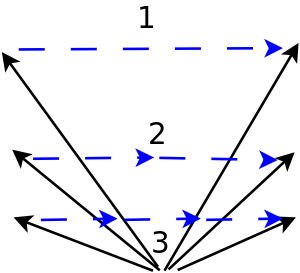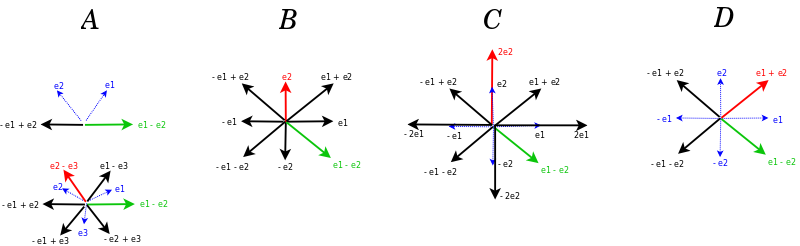Literature

Video'

• Hermitian K-theory by Karoubi is an analogue to Lie classical groups - orthogonal, unitary, symplectic - and perhaps a unification of them. See Karoubi's video 32:00 on Quadratic forms and Bott periodicity.

#### ClassicalLieGroups

Naujausi pakeitimai

 Puslapis paskutinį kartą pakeistas 2019 gegužės 14 d., 17:17